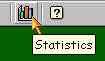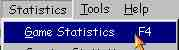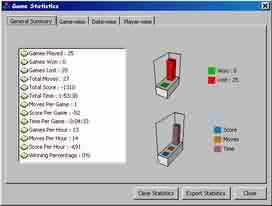# Solitaire Game Statistics Window

Game Statistics window gives you statistics of played games by all active players exists in the game's player subsystem. To open game statistics window click on "Statistics" button on the toolbar.or click on Game Statistics under Statistics menu or press F4This will open the game statistics Window.Left side of the window gives a listing of all the statistics like Total games played, won, lost, total moves, score, time, moves per game, score per game, time per game, games per hour, score per hour and winning percentage. Right side of the window shows two charts one is for won/lost and other is score/moves/time chart. These statistics and charts are very useful because it gives you an overall picture of the total statistics. A move is a simple card movement so total moves is the total cards moves so far and moves per game is the average card movements. Score is dependent on cards moved and time taken to move those cards. Total score is the sum of scores achieved in all played games including incomplete games. Score per game is the average score achieved i.e. total score divided by total games played. Time per game is the average time taken to complete a game (either win or loss). Games per hour gives an average of games played in each hour. This is calculated by total games played divided by total time played. similarly we calculate score per hour and moves per hour. Winning percentage gives an idea of your overall performance. It is calculated in this way. Winning Percentage= (Total Games Won/Total Games Played) * 100 All our games have options to clear the statistics. When you clear or erase all statistics then game statistics window values also be erased and will start from the beginning.